Function Repository Resource:

# ConjugatePartition

Switch the rows and columns of a partition

Contributed by: Wolfram Staff
 ResourceFunction["ConjugatePartition"][p] gives the partition that transposes the rows and columns of the integer partition p.

## Details

A partition of n is a list of weakly decreasing positive integers that add up to n. For instance, written compactly, these are the five partitions of 4: 4, 31, 22, 211, 1111.

## Examples

### Basic Examples (2)

A partition of 10 and its conjugate:

 In:=Out=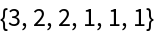Use the resource function FerrersDiagram to show the Ferrers diagrams of the partition and its conjugate together:

 In:=Out=Some partitions are self-conjugate:

 In:=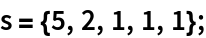In:=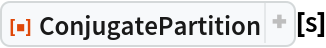Out=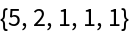Using the resource function FerrersDiagram, verify that a self-conjugate partition has a symmetric Ferrers diagram:

 In:=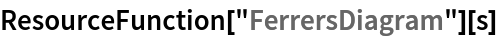Out=George Beck

## Version History

• 1.0.1 – 31 January 2022
• 1.0.0 – 31 May 2019